# Piezo Bender

Piezoelectric bimorph beam of rectangular cross-section

• Library:
• Simscape / Electrical / Electromechanical / Mechatronic Actuators

•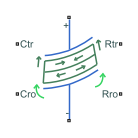## Description

The Piezo Bender block models a piezoeletric bimorph beam of rectangular cross-section.

A piezo bender is a piezoelectric device that bends when you apply an electrical potential between its plates. Conversely, when a piezo bender bends, it generates an electrical potential.

A piezo bender comprises different rectangular layers of piezoelectic material with a polarization perpendicular to the stack. This polarization is alternated in each layer.

### Equations

This figure shows the cartesian reference frame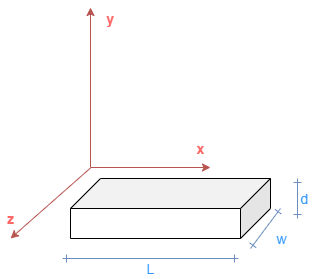where:

• L is the beam length.

• w is the beam width.

• d is the beam thickness.

These are the constitutive equations for a piezoelectric material in the stress-charge formulation,

 $T=\left[c\right]S-{\left[e\right]}^{T}E$ (1)
 $D=\left[e\right]S-\left[{ϵ}^{S}\right]E$ (2)

where:

• T is the stress field.

• [c] is the compliance tensor.

• S is the strain field.

• [e] is the piezo stress coefficient tensor.

• E is the electric field.

• D is the electric displacement field.

• S] is the permittivity tensor at constant or zero strain.

To model the flexibility, the block uses the Euler-Bernoulli finite-element beam equations. The translation and rotation of each beam cross-section in function of the x-axis determine the beam kinematics.

This block considers only the forces applied in the y direction and the piezoelectric material is polarized to bend only in the x-y plane. For this reason, to describe the kinematics, you only need to specify the vertical displacement in the y direction of the center of gravity of each cross-section, y(x), and the rotation around the z-axis of each cross-section, φz(x).

From the previous assumptions, the strain field in an Euler-Bernoulli beam subject to bending is equal to:

 ${S}_{xx}\left(x,y\right)=-y\frac{d{\phi }_{z}}{dx}\left(x\right).$ (3)

Because the electric field is constant between the positive and negative plates, ${E}_{y}=\frac{v}{d}$, the block substitutes equation 3 into equation 1:

`${T}_{xx}\left(x,y\right)=-Ey\frac{d{\phi }_{z}}{dx}\left(x\right)-{e}_{31}\frac{v}{d}.$`

In this equation, E = c11 is the Young modulus of the material and e31 is the (3,1) piezoelectric stress-charge coupling coefficient, ${\sigma }_{xx}=-{e}_{31}{E}_{y}$.

This equation defines the bending moment from the stress field:

`${M}_{z}\left(x\right)=-\iint y{T}_{xx}\left(x,y\right)\text{\hspace{0.17em}}dS=\iint E{y}^{2}\frac{d{\phi }_{z}}{dx}\left(x\right)+{e}_{31}\frac{v}{d}y\text{\hspace{0.17em}}dS.$`

Because the polarization of the material for $y=\left[-\frac{d}{2},0\right]$ is the opposite than the polarization for $y=\left[0,\frac{d}{2}\right]$, the (3,1) piezoelectric stress-charge coupling coefficient changes sign and the bending moment is defined by

`${M}_{z}\left(x\right)=\frac{E}{12}w\text{\hspace{0.17em}}{d}^{3}\frac{d{\phi }_{z}}{dx}\left(x\right)+w\text{\hspace{0.17em}}d\text{\hspace{0.17em}}{e}_{31}v=EI\frac{d{\phi }_{z}}{dx}\left(x\right)+w\text{\hspace{0.17em}}d\text{\hspace{0.17em}}{e}_{31}v,$`

where $I=\frac{1}{12}w{d}^{3}$ is the second moment of area of the rectangular cross-section.

In this equation, the first term is the classical equation of a beam subject to bending, and the second term is the electromechanical coupling due to the presence of a voltage across the piezoelectric material. This voltage produces a uniform electrical bending moment loaded along the beam.

The block then substitutes equation 3 into equation 2:

`${D}_{y}\left(x,y\right)=-{e}_{31}y\frac{d{\phi }_{z}}{dx}\left(x\right)+\frac{ϵv}{d}.$`

The electric charge inside a volume is equal to the Gauss integral of the electric displacement:

`$dq\left(x\right)=∯{D}_{y}\text{\hspace{0.17em}}dS={e}_{31}dw\text{\hspace{0.17em}}dx\frac{d{\phi }_{z}}{dx}={e}_{31}dw\text{\hspace{0.17em}}d{\phi }_{z}.$`

Then, this equation defines the charge accumulated between the two sections of the beam due to the piezoelectric effect:

`$q={e}_{31}dw\left({\phi }_{z}\left({x}_{2}\right)-{\phi }_{z}\left({x}_{1}\right)\right).$`

Finally, from the mechanical perspective, you can model a piezo bender as an Euler-Bernoulli beam loaded with a uniform torque that is proportional to the voltage:

`${M}_{z}\left(x\right)=EI\frac{d{\phi }_{z}}{dx}\left(x\right)+w\text{\hspace{0.17em}}d\text{\hspace{0.17em}}{e}_{31}v$`

From the electrical perspective, you can model a piezo bender as a capacitor with a charge source proportional to the bending angle:

`$q={e}_{31}d\text{\hspace{0.17em}}w\left({\phi }_{zR}-{\phi }_{zC}\right)+\frac{ϵwl}{d}v.$`

### Finite Element Formulation

To discretize and solve the Euler-Bernoulli equations with the piezoelectric coupling, the Piezo Bender block uses a Finite Element method.

The block discretizes the piezo bender beam into a number of slices in the length direction with the same width, w, and thickness, d. The length of each element is equal to the total length of the beam divided by the number of elements, $l=\frac{L}{{N}_{elements}}$.

This stiffness matrix of a finite element of an Euler-Bernoulli beam defines the relationship between the vertical displacement and rotational angle of each end of the beam element, and the corresponding forces and moments due to the beam elasticity:

`$\left[\begin{array}{c}{F}_{C}\\ {T}_{C}\\ {F}_{R}\\ {T}_{R}\end{array}\right]=\left[\begin{array}{cccc}\frac{12EI}{{l}^{3}}& \frac{6EI}{{l}^{2}}& -\frac{12EI}{{l}^{3}}& \frac{6EI}{{l}^{2}}\\ \frac{6EI}{{l}^{2}}& \frac{4EI}{l}& -\frac{6EI}{{l}^{2}}& \frac{2EI}{l}\\ -\frac{12EI}{{l}^{3}}& -\frac{6EI}{{l}^{2}}& \frac{12EI}{{l}^{3}}& -\frac{6EI}{{l}^{2}}\\ \frac{6EI}{{l}^{2}}& \frac{2EI}{l}& -\frac{6EI}{{l}^{2}}& \frac{4EI}{l}\end{array}\right]\left[\begin{array}{c}{y}_{C}\\ {\phi }_{C}\\ {y}_{R}\\ {\phi }_{R}\end{array}\right].$`

Then, to obtain the equations for a piezo bender beam element, add the coupling terms and the mass matrix for the inertia:

`$\frac{\rho lwd}{420}\left[\begin{array}{cccc}156& 22l& 54& -13l\\ 22l& 4{l}^{2}& 13l& -3{l}^{2}\\ 54& 13l& 156& -22l\\ -13l& -3{l}^{2}& -22l& 4{l}^{2}\end{array}\right]\left[\begin{array}{c}\frac{{d}^{2}{y}_{C}}{d{t}^{2}}\\ \frac{{d}^{2}{\phi }_{C}}{d{t}^{2}}\\ \frac{{d}^{2}{y}_{R}}{d{t}^{2}}\\ \frac{{d}^{2}{\phi }_{R}}{d{t}^{2}}\\ 0\end{array}\right]+\left[\begin{array}{ccccc}\frac{12EI}{{l}^{3}}& \frac{6EI}{{l}^{2}}& -\frac{12EI}{{l}^{3}}& \frac{6EI}{{l}^{2}}& 0\\ \frac{6EI}{{l}^{2}}& \frac{4EI}{l}& -\frac{6EI}{{l}^{2}}& \frac{2EI}{l}& {e}_{31}wd\\ -\frac{12EI}{{l}^{3}}& -\frac{6EI}{{l}^{2}}& \frac{12EI}{{l}^{3}}& -\frac{6EI}{{l}^{2}}& 0\\ \frac{6EI}{{l}^{2}}& \frac{2EI}{l}& -\frac{6EI}{{l}^{2}}& \frac{4EI}{l}& -{e}_{31}wd\\ 0& -{e}_{31}wd& 0& {e}_{31}wd& \frac{ϵwl}{d}\end{array}\right]\left[\begin{array}{c}{y}_{C}\\ {\phi }_{C}\\ {y}_{R}\\ {\phi }_{R}\\ v\end{array}\right]=\left[\begin{array}{c}{F}_{C}\\ {T}_{C}\\ {F}_{R}\\ {T}_{R}\\ q\end{array}\right].$`

Finally, this is the equation for a piezo bender beam element with damping:

`$\frac{\rho lwd}{420}\left[\begin{array}{cccc}156& 22l& 54& -13l\\ 22l& 4{l}^{2}& 13l& -3{l}^{2}\\ 54& 13l& 156& -22l\\ -13l& -3{l}^{2}& -22l& 4{l}^{2}\end{array}\right]\left[\begin{array}{c}\frac{{d}^{2}{y}_{C}}{d{t}^{2}}\\ \frac{{d}^{2}{\phi }_{C}}{d{t}^{2}}\\ \frac{{d}^{2}{y}_{R}}{d{t}^{2}}\\ \frac{{d}^{2}{\phi }_{R}}{d{t}^{2}}\\ 0\end{array}\right]+\left[\begin{array}{ccc}\left[B\right]& \cdots & 0\\ ⋮& \ddots & ⋮\\ 0& \cdots & 0\end{array}\right]\left[\begin{array}{c}\frac{d{y}_{C}}{dt}\\ \frac{d{\phi }_{C}}{dt}\\ \frac{d{y}_{R}}{dt}\\ \frac{d{\phi }_{R}}{dt}\\ 0\end{array}\right]+\left[\begin{array}{ccccc}\frac{12EI}{{l}^{3}}& \frac{6EI}{{l}^{2}}& -\frac{12EI}{{l}^{3}}& \frac{6EI}{{l}^{2}}& 0\\ \frac{6EI}{{l}^{2}}& \frac{4EI}{l}& -\frac{6EI}{{l}^{2}}& \frac{2EI}{l}& {e}_{31}wd\\ -\frac{12EI}{{l}^{3}}& -\frac{6EI}{{l}^{2}}& \frac{12EI}{{l}^{3}}& -\frac{6EI}{{l}^{2}}& 0\\ \frac{6EI}{{l}^{2}}& \frac{2EI}{l}& -\frac{6EI}{{l}^{2}}& \frac{4EI}{l}& -{e}_{31}wd\\ 0& -{e}_{31}wd& 0& {e}_{31}wd& \frac{ϵwl}{d}\end{array}\right]\left[\begin{array}{c}{y}_{C}\\ {\phi }_{C}\\ {y}_{R}\\ {\phi }_{R}\\ v\end{array}\right]=\left[\begin{array}{c}{F}_{C}\\ {T}_{C}\\ {F}_{R}\\ {T}_{R}\\ q\end{array}\right]$`

where:

• l is the element length.

• w is the element width.

• d is the element thickness.

• $I=\frac{1}{12}w{t}^{3}$ is the second moment of area.

• E is the Young modulus.

• m = ρlwd is the element mass, where ρ is the mass density.

• e31 is the (3,1) piezoelectric stress-charge coupling coefficient, ${\sigma }_{xx}=-{e}_{31}{E}_{y}$.

• ε is the electrical permittivity.

• $B={b}_{k}K+{b}_{m}M$ is the damping matrix.

• bm is the Rayleigh damping coefficient proportional to mass.

• bk is the Rayleigh damping coefficient proportional to stiffness.

• $K=\left[\begin{array}{cccc}\frac{12EI}{{l}^{3}}& \frac{6EI}{{l}^{2}}& -\frac{12EI}{{l}^{3}}& \frac{6EI}{{l}^{2}}\\ \frac{6EI}{{l}^{2}}& \frac{4EI}{l}& -\frac{6EI}{{l}^{2}}& \frac{2EI}{l}\\ -\frac{12EI}{{l}^{3}}& -\frac{6EI}{{l}^{2}}& \frac{12EI}{{l}^{3}}& -\frac{6EI}{{l}^{2}}\\ \frac{6EI}{{l}^{2}}& \frac{2EI}{l}& -\frac{6EI}{{l}^{2}}& \frac{4EI}{l}\end{array}\right]$ is the stiffness finite element matrix.

• $M=\frac{\rho lwd}{420}\left[\begin{array}{cccc}156& 22l& 54& -13l\\ 22l& 4{l}^{2}& 13l& -3{l}^{2}\\ 54& 13l& 156& -22l\\ -13l& -3{l}^{2}& -22l& 4{l}^{2}\end{array}\right]$ is the mass matrix.

• yC is the deflection along the y-axis of the left-end of the element.

• yR is the deflection along the y-axis of the right-end of the element.

• φC is the rotation around the z-axis of the left-end of the element.

• φR is the rotation around the z-axis of the right-end of the element.

• FC is the force along the y-axis of the left-end of the element.

• FR is the force along the y-axis of the right-end of the element.

• TC is the torque in the z-axis of the left-end of the element.

• TR is the torque in the z-axis of the right-end of the element.

• v is the voltage across the top and bottom electrodes.

• q is the accumulated charge between the electrodes and the piezoelectric material.

### Datasheet Parameterization

A datasheet of a piezo bender usually provides this data:

• Dimensions (l, w, d)

• Mass, m

• Rated voltage, vrated

• Free deflection at rated voltage, yfree

• Blocking force at rated voltage, Fblock

• Capacitance, Cpiezo

• First resonant frequency, f1

It is possible to calculate the fundamental material parameters of a piezo bender by using the datasheet parameters.

First, the block solves the voltage-force deflection relationships from the steady-state equations with no torque applied and clamped-free configuration:

`$\left[\begin{array}{ccc}\frac{12EI}{{l}^{3}}& -\frac{6EI}{{l}^{2}}& 0\\ -\frac{6EI}{{l}^{2}}& \frac{4EI}{l}& -{e}_{31}w\text{\hspace{0.17em}}d\\ 0& {e}_{31}w\text{\hspace{0.17em}}d& \frac{\epsilon \text{\hspace{0.17em}}wl}{d}\end{array}\right]\left[\begin{array}{c}{y}_{R}\\ {\phi }_{R}\\ v\end{array}\right]=\left[\begin{array}{c}{F}_{R}\\ 0\\ q\end{array}\right].$`

These equations define the relationship between the tip deflection, voltage and tip force:

`$\begin{array}{l}{y}_{R}=\frac{{l}^{2}\left(4{F}_{R}\text{\hspace{0.17em}}l+6\text{\hspace{0.17em}}d\text{\hspace{0.17em}}w\text{\hspace{0.17em}}{e}_{31}\text{\hspace{0.17em}}v\right)}{12EI}=\frac{{l}^{2}\text{\hspace{0.17em}}\left(4{F}_{R}\text{\hspace{0.17em}}l+6\text{\hspace{0.17em}}d\text{\hspace{0.17em}}w\text{\hspace{0.17em}}{e}_{31}\text{\hspace{0.17em}}v\right)}{E\text{\hspace{0.17em}}w\text{\hspace{0.17em}}{d}^{3}}={F}_{R}\frac{4{l}^{3}}{Ew{d}^{3}}+v\frac{6\text{\hspace{0.17em}}{e}_{31}{l}^{2}}{E\text{\hspace{0.17em}}{d}^{2}}\\ {y}_{free}=\frac{6{l}^{2}\text{\hspace{0.17em}}d\text{\hspace{0.17em}}w\text{\hspace{0.17em}}{e}_{31}\text{\hspace{0.17em}}{v}_{rated}}{12EI}=\frac{6\text{\hspace{0.17em}}{e}_{31}{l}^{2}\text{\hspace{0.17em}}{v}_{rated}}{E\text{\hspace{0.17em}}{d}^{2}}\\ {F}_{block}=-\frac{3\text{\hspace{0.17em}}d\text{\hspace{0.17em}}w\text{\hspace{0.17em}}{e}_{31}\text{\hspace{0.17em}}{v}_{rated}}{2l}\end{array}$`

The block computes the capacitance by assuming zero applied force:

`${C}_{piezo}=\epsilon \frac{l\text{\hspace{0.17em}}w}{d}+{e}_{31}^{2}\frac{l\text{\hspace{0.17em}}{d}^{2}{w}^{2}}{EI}=\epsilon \frac{l\text{\hspace{0.17em}}w}{d}+{e}_{31}^{2}\frac{12l\text{\hspace{0.17em}}w}{Ed}=\left(\epsilon +12\frac{{e}_{31}^{2}}{E}\right)\frac{l\text{\hspace{0.17em}}w}{d}.$`

Finally, this equation shows the relationship between density and mass:

`$m=\rho \text{\hspace{0.17em}}lwd.$`

Once you have defined all the relationships between the fundamental and datasheet parameters, you can calculate the fundamental parameters with these equations:

`$\begin{array}{l}{e}_{31}=-\frac{2\text{\hspace{0.17em}}l\text{\hspace{0.17em}}{F}_{block}}{3\text{\hspace{0.17em}}d\text{\hspace{0.17em}}w\text{\hspace{0.17em}}{v}_{rated}}\\ E=-\frac{4\text{\hspace{0.17em}}{F}_{block}{l}^{3}}{{y}_{free}{d}^{3}w}\\ \epsilon =\frac{d}{l\text{\hspace{0.17em}}w}\left({C}_{piezo}+\frac{4{F}_{block}{y}_{free}}{{v}_{rated}^{2}}\right)\end{array}$`

Then substitute these equations in the constitutive equations:

`$\begin{array}{l}\frac{v}{{v}_{rated}}=\frac{{F}_{R}}{{F}_{block}}+\frac{{y}_{R}}{{y}_{free}}\\ q={C}_{piezo}v+\frac{{y}_{free}}{{v}_{rated}}{F}_{R}\end{array}$`

Dynamics Datasheet Parameterization

You can calculate the first resonance frequency of a clamped-free beam of uniform cross section by using this equation:

`$2\pi {f}_{0}={1.855}^{2}\sqrt{\frac{EI}{m\text{\hspace{0.17em}}{l}^{3}}}.$`

The block then parameterizes the dynamics directly by specifying the desired natural frequency.

### Boundary Conditions

Beams have different boundary conditions at their left and right ends:

• Free — Both the displacement and rotation are equal to any value.

• Simply supported — The displacement is equal to `0`.

• Clamped — Both the displacement and rotation are equal to `0`.

This table shows the possible boundary configurations for a piezo bender beam.

ConfigurationModel
Clamped-free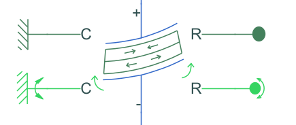Supported-supported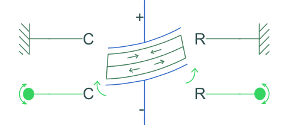Clamped-clamped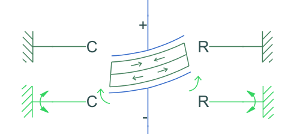## Ports

### Conserving

expand all

Electrical conserving port associated with the piezo bender positive terminal.

Electrical conserving port associated with the piezo bender negative terminal.

Mechanical translational conserving port associated with the case.

Mechanical rotational conserving port associated with the case.

Mechanical translational conserving port associated with the rotor.

Mechanical rotational conserving port associated with the rotor.

## Parameters

expand all

### Dimensions

Number of elements in which the piezo bender beam is discretized using the Euler-Bernoulli finite elements equations.

Total length of the beam.

Width of the beam.

Thickness of the beam.

Whether to parameterize the steady state parameters from a datasheet or from material properties.

Capacitance of the piezo bender.

#### Dependencies

To enable this parameter, set Parameterization to ```Specify from a datasheet for clamped-free configuration```.

Rated drive voltage.

#### Dependencies

To enable this parameter, set Parameterization to ```Specify from a datasheet for clamped-free configuration```.

Tip deflection when no force is applied. The sign of this parameter must be different from the sign of the Blocking force at Vrated parameter.

#### Dependencies

To enable this parameter, set Parameterization to ```Specify from a datasheet for clamped-free configuration```.

Force that nullifies the tip deflection. The sign of this parameter must be different from the sign of the Free deflection at Vrated parameter.

#### Dependencies

To enable this parameter, set Parameterization to ```Specify from a datasheet for clamped-free configuration```.

Young modulus, or the modulus of elasticity in tension.

#### Dependencies

To enable this parameter, set Parameterization to ```Specify from material properties```.

(3,1) piezoelectric stress-charge coupling coefficient.

#### Dependencies

To enable this parameter, set Parameterization to ```Specify from material properties```.

Dielectric constant that represents the relative permittivity of the material. This parameter must be bigger than the vacuum permittivity.

#### Dependencies

To enable this parameter, set Parameterization to ```Specify from material properties```.

### Dynamics

Whether to model inertial effects.

Whether to parameterize the inertia parameters from a datasheet or from material properties.

#### Dependencies

To enable this parameter, set Inertial effects to `On`.

Mass of the beam.

#### Dependencies

To enable this parameter, set Inertial effects to `On` and Parameterization to ```Specify from material properties```.

First natural frequency.

#### Dependencies

To enable this parameter, set Inertial effects to `On` and Parameterization to ```Specify from a datasheet for clamped-free configuration```.

Damping coefficient proportional to stiffness, for Rayleigh damping.

Damping coefficient proportional to mass, for Rayleigh damping.

#### Dependencies

To enable this parameter, set Inertial effects to `On`.

 Tadmor, E. B., and G. Kosa. "Electromechanical Coupling Correction for Piezoelectric Layered Beams." Journal of Microelectromechanical Systems, vol. 12, no. 6, Dec. 2003, pp. 899–906. DOI.org (Crossref), doi:10.1109/JMEMS.2003.820286.

 Benjeddou A, Trindade MA, Ohayon R. "A Unified Beam Finite Element Model for Extension and Shear Piezoelectric Actuation Mechanisms." Journal of Intelligent Material Systems and Structures. 1997;8(12):1012-1025. doi:10.1177/1045389X9700801202

 Gavin, Henri P. "Beam Element Stiﬀness Matrices." CEE 421L. Matrix Structural Analysis. Duke University, 2014.